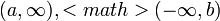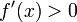# Positive derivative implies increasing

(diff) ← Older revision | Latest revision (diff) | Newer revision → (diff)

## Statement

### On an open interval

Suppose$f$ is a function on an open interval$I$ that may be infinite in one or both directions (i..e,$I$ is of the form$(a,b)$,$(a,\infty), [itex](-\infty,b)$, or$(-\infty,\infty)$). Suppose the derivative of$f$ exists and is positive everywhere on$I$, i.e.,$f'(x) > 0$ for all$x \in I$. Then,$f$ is an increasing function on$I$, i.e.:$x_1, x_2 \in I, \qquad x_1 < x_2 \implies f(x_1) < f(x_2)$

## Facts used

1. Lagrange mean value theorem

## Proof

Fill this in later